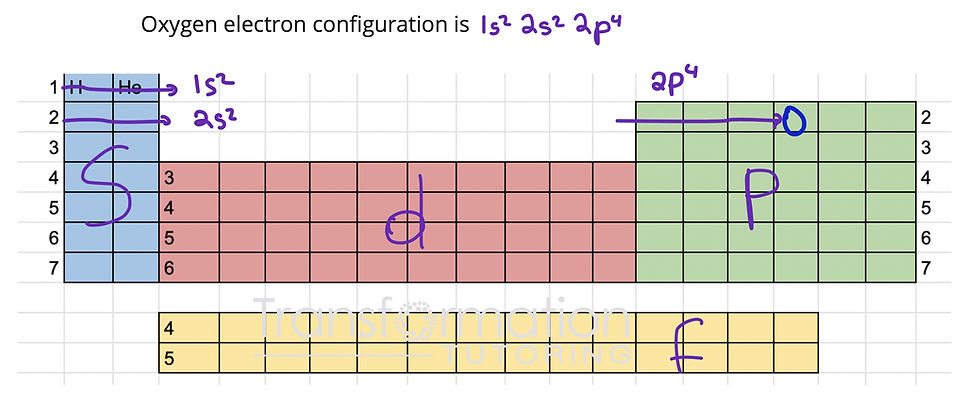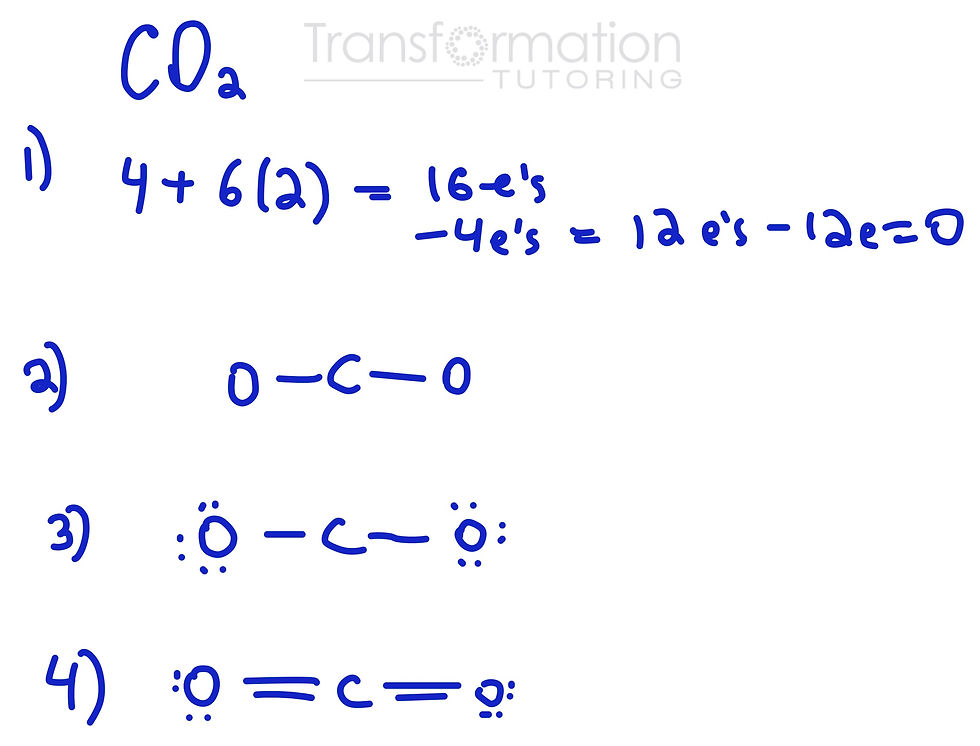top of page
Search

# Organic Chemistry by Brown, Iverson, Anslyn, Foote | Mayya's Study Guide| Chapter 1

Chapter 1: Covalent Bonding And Shapes Of Molecules

• Electron Configuration

• Orbital Energy Diagram

• Lewis Dot Structures

• Shapes of Molecules and Their Bond Angles

• Formal Charge

• Functional Groups

• Hybridization

• Resonance

Electron Configuration

Step 1: Divide Periodic Table into s, p, d and f blocks as shown. Move helium next to hydrogen.

Step 2: Number the rows on the Periodic Table on the left and right sides. Number the d block 1 less then S block as shown. Number f block as well.Step 3: Locate the element on the periodic table. Travel starting from the top left to the right through each row until you reach the element needed. As you travel through the table, write out the electron configuration. For example, as you travel though the first row, you will get 1s^2. 1 is the number of the row, s is the name of the block and the superscript 2 is the number of squares in the block you are getting through.

Let's try some examples:

Oxygen electron configurationAs I go through the first row, I get 1s2. Then, I have to start at the second row and I get 2s2, followed by 2p4 (since oxygen is in the fourth square on the p block row 2).

We can also write out the electron configuration as a noble gas configuration. All we need to do is find the noble gas (Group 18 on the very right) that is before our element. We put that noble gas into brackets and follow by writing the rest of the electron configuration. For oxygen, we would have [He] 2s^2 2p^4.

Note: In the textbook, the authors breakdown p sub shell into 3 orbitals: px, py and pz. Instead of writing 2p4 for oxygen, we would write 2px^2 2py^1 2pz^1. More on electron distribution in the part below.

Bromine electron configurationbromine electron configuration using period table

Orbital Energy Diagram

Follow electron configuration to write out the orbital diagram.

S subshell has one orbital. P has 3 orbitals.

D has 5 orbitals and f has 7 orbitals. Each orbital has a maximum of 2 electrons. We represent orbitals by dashes and electrons by arrows.

Rules:

1. Follow electron configuration filling out orbitals from lowest energy to highest: Aufbau Rule

2. In the same subshell, first distribute electrons one in each orbital with parallel spins before pairing them: Hund's Rule

Let's show orbital energy diagram for chlorinechlorine orbital diagram

Lewis Dot Structures

Mayya's Tip:

1. Calculate the total number of valence electrons in a molecule (group number = number of valence electrons)

2. Draw our your molecule and put lines between atoms (bonds)

3. Subtract electrons used in bonds (2 electrons per bond) from the total number of valence electrons

4. Use the remaining electrons to make outer atoms happy (octet). Keep in mind hydrogen only wants 2 electrons.

5. If there are any electrons left, place them on the central atom.

6. Check if everyone is happy(octet). If not use neighboring atom's lone pairs to make double and triple bonds.

Lewis Dot Structure for CO2how to draw CO2 lewis dot structure

Shapes of Molecules and Their Bond AnglesWe need to use this chart and memorize it to determine the molecular geometry/shape of a molecule. First, we must start with the correct Lewis Dot Structure. Next, we have to find the central atom and identify how many other atoms it is attached to and how many lone pairs it has. In the chart, the purple dot represents the central atom, blue dots are atoms the central atom is attached to and two dots represent a lone electron pair.

For example, in CO2 example above, carbon is the central atom that is attached to two other atoms (oxygens) and has no lone pairs. If we check our chart, we see that case on the top right, making this molecule linear. The bond angle would be 180 degrees according to the chart.

Formal Charge

Formal Charge = number of valence electrons - (number of bonds + number of lone electrons)Formal Charge for HCO3-

Let's take a look at the example above and calculate the formal charge for the oxygen on the right. Oxygen has 6 valence electrons (it's in group 6 on the periodic table). We subtract from it the number of bonds it has (1) and the number of lone electrons it has (6). 6-(1+6) = -1

Functional Groupsfunctional groups organic chemistry

Hybridization

Steric Number = number of attachments + number of lone pairsHow to determine hybridization of an atom

We can use the chart above to determine hybridization of an atom in a molecule. For example, let's take a look at CO2 we have shown above and determine hybridization of carbon. The steric number for carbon is 2 because it is attached to two other atoms and has no lone pairs. According to the chart, hybridization is sp when steric number is 2.